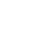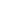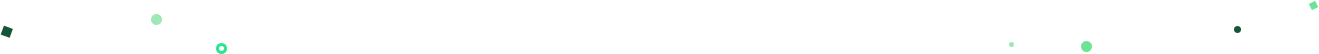Introducing Visual Explorer, a new tool for data visualization.Learn more## Mode Studio

The Collaborative Data Science Platform

# SQL WHERE

Starting here? This lesson is part of a full-length tutorial in using SQL for Data Analysis. Check out the beginning.

In this lesson we'll cover:

## The SQL WHERE clause

Start by running a SELECT statement to re-familiarize yourself with the housing data used in this tutorial. Remember to switch over to Mode and run any of the code you see in the light blue boxes to get a sense of what the output will look like.

``````SELECT * FROM tutorial.us_housing_units
``````

Once you know how to view some data using `SELECT` and `FROM`, the next step is filtering the data using the `WHERE` clause. Here's what it looks like:

``````SELECT *
FROM tutorial.us_housing_units
WHERE month = 1
``````

Note: the clauses always need to be in this order: `SELECT`, `FROM`, `WHERE`.

## How does WHERE work?

The SQL `WHERE` clause works in a plain-English way: the above query does the same thing as `SELECT * FROM tutorial.us_housing_units`, except that the results will only include rows where the `month` column contains the value `1`.

In Excel, it's possible to sort data in such a way that one column can be reordered without reordering any of the other columns—though that could badly scramble your data. When using SQL, entire rows of data are preserved together. If you write a `WHERE` clause that filters based on values in one column, you'll limit the results in all columns to rows that satisfy the condition. The idea is that each row is one data point or observation, and all the information contained in that row belongs together.

You can filter your results in a number of ways using comparison and logical operators, which you'll learn about in the next lesson.

Next Lesson

SQL Comparison Operators# Get our weekly data newsletter

Work-related distractions for every data enthusiast.